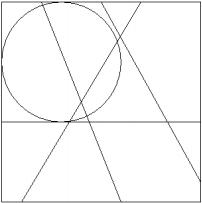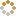﻿ Ningbo University of Technology Online Judge ::  Pieces and Discs
• ###  Pieces and Discs

• 时间限制: 1000 ms　内存限制: 65535 K
• 问题描述
• There is a rectangle on the Cartesian plane, whose bottom-left corner is (0,0), top-right corner is (L,W). You draw some line segments to divide the rectangle into pieces. Each line segment connects two points on the boundary of the original rectangle (these two points are guaranteed to be on different sides of the rectangle).

Finally, you draw some discs (a disc is a circle with its interior), and your task is to find out all the pieces each disc is intersecting with (i.e. pieces that have non-zero intersection area with the disc), and output their areas in increasing order.

An example picture is shown below:• 输入
• There will be at most 100 test cases. Each test case begins with four integer n, m, L, W (1<=n,m<=20, 1<=L,W<=100), where n is the number of line segments, m is the number of discs. Each of the next n lines describes a line segment with four integers x1, y1, x2, y2, that is a segment connecting (x1,y1) and (x2,y2). These two points are guaranteed to be on different sides of the original rectangle. Each of the last m line contains three integers x, y, R (0<=x<=L, 0<=y<=W, 1<=R<=100),indicating that the disc is centered at (x, y), whose radius is R. No two segments will be the same. Input is terminated by n=m=L=W=0.
• 输出
• For each disc (same order as in input), print the number of pieces that the disc is intersecting with, and the areas of these pieces in a single line. The areas should be sorted in increasing order, and each area should be rounded to 2 digits after the decimal point. <strong>Print a blank line after each test case. </strong>
• 样例输入
• ```4 1 10 10
0 4 10 4
1 0 7 10
5 10 10 1
2 10 6 0
3 7 3
0 0 0 0
```
• 样例输出
• `4 0.50 10.03 10.77 18.70`
• 提示
• `无`
• 来源
• `The Seventh Hunan Collegiate Programming Contest`
• 操作
﻿